# Velocity - 8th grade (13y) - math problems

The velocity of an object is the time rate of change of its position. Velocity is a specification of an object's speed and direction of motion (e.g. 20 m/s to the south).

In everyday use and in kinematics, the speed of an object is the magnitude of its velocity (the rate of change of its position) - scalar.

#### Number of problems found: 198

• MinuteTwo boys started from one place. First went north at velocity 3 m/s and the second to the east with velocity 4 m/s. How far apart they are after minute?
• PumpsPump that draws water at velocity 3.5 liters per second water from a construction trench take 35 minutes. a) Find out how many minutes the water would run out of the trench pump that draws 7.4 liters of water per second. b) What is the pumping velocity wo
• Positional energyWhat velocity in km/h must a body weighing 60 kg have for its kinetic energy to be the same as its positional energy at the height 50 m?
• An airplaneAn airplane flies 1440 km in 2 1/4 hours. What is its average speed in km per hour?
• Wave parameters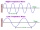Calculate the speed of a wave if the frequency is 336 Hz and the wavelength is 10 m.
• Working togetherOne worker performs work in 3 days, the second in 5 days. How much do they work together?
• RPMAn electric motor makes 3,000 revolutions per minutes. How many degrees does it rotate in one second?
• Bob traveled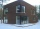Bob traveled 20 meters to Dave's house. He arrived in 3 minutes. What was his average speed for the trip.
• Magnitude of angleWhat magnitude has an obtuse angle enclosed by the hands of clocks at 12:20 hours?
• Mail trainThe speed of mail train is 1370 meter per minute. Express it in miles per hour correct to three significant figure . Given that 1 meter =39.37 inches.
• A bus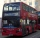A bus is scheduled to travel a distance 121 km in 2 3/4 hours. What average rate of speed must be maintained to arrive on schedule?
• Two boats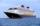The sightseeing boat sail at 25 km / h. The cruise take 6 hours. How long the another boat sails at 60 km/h?
• Saw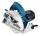Blade circular saw with a diameter 42 cm turns 825 times per minute. Expresses his cutting speed in meters per minute.
• 3 pupils3 pupils cleaned 10 meters of the stream in 1 hour. How many meters of the stream will clean 18 students in 4 hours?
• The fastestThe fastest boat can reach speeds more than 710% as fast as the Queen Mary 2. How would you express this number as a fraction and as a decimal?
• Two trains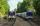Two trains go at each other. First at speed 40 km/h and the second at speed 60 km/h. At what distance will be 30 minutes before they will meet?
• Cable carThe cable car will carry 1280 passengers in 40 minutes. How many people will be transported in 2.5 hours?
• DoublingThe message is spreading that each day has doubled the number of people who know about it. All know message for 20 days. How long known it eighth people?
• ClockHow many times a day hands on a clock overlap?
• Timber logThe ship goes from point x to y. Downstream it takes 4 hours, upstream 6 hours. How long does it take from a point x to y a log?

Do you have an interesting mathematical word problem that you can't solve it? Submit a math problem, and we can try to solve it.

We will send a solution to your e-mail address. Solved examples are also published here. Please enter the e-mail correctly and check whether you don't have a full mailbox.

Please do not submit problems from current active competitions such as Mathematical Olympiad, correspondence seminars etc...